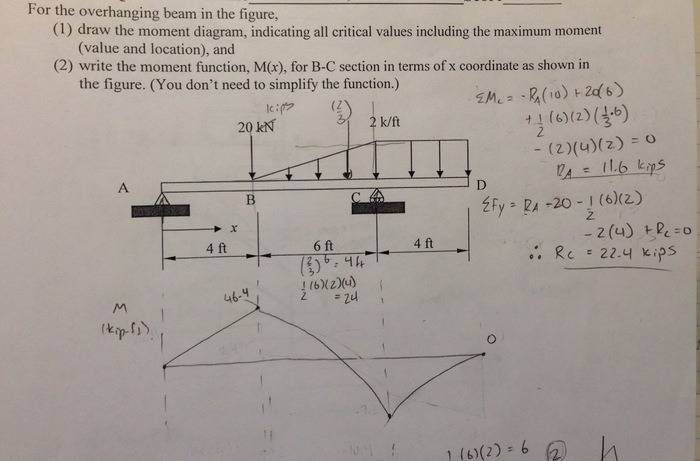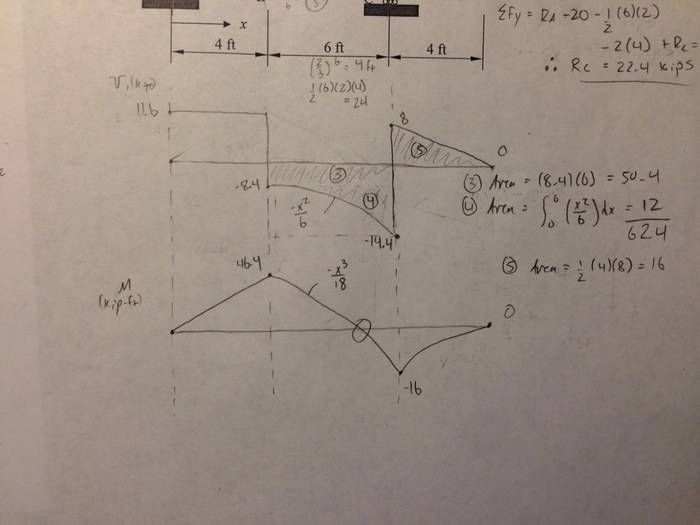# Moment diagram with triangular load

## Homework Statement

For the overhanging beam in the figure, A) draw the moment diagram indicating all critical values including the maximum moment (Value and location), and B) write the moment function, M(x), for B-C section in terms of x coordinate as shown in the figure.

## Homework Equations

F = ma
M = F*d
(1/3x) <---- (Equation for the slope of the triangular load from B-C I think)
-1/6x^2 <---- (Equation for the slope of the shear load from B-C I think)
-1/18x^3 <---- (Equation for the slope of the moment diagram from B-C I think)

## The Attempt at a Solution

Mc = -Ra(10) + (20)(6) + 1/2(6)(2)(1/3(6)) - (2)(4)(2) = 0 therefore, Ra = 11.6 kips
Fy = Ra - 20 - 1/2(6)(2) - 2(4) + Rc = 0 therefore, Rc = 22.4 kips

I'm trying to figure out how to find where the middle cubic function crosses the x-axis and I'm having a really hard time. Could I get a push in the right direction please?SteamKing
Staff Emeritus
Homework Helper
Step 1: Figure out the reactions at A and C.
Step 2: Construct the shear force diagram for the beam with these reactions.
Step 3: Using the shear force diagram, construct the bending moment diagram.

You are trying to construct the moment diagram by jumping in the middle of the process without completing the basic steps (1 and 2 above) first.

Alrighty thanks for the reply SteamKing, I did as you described and did the shear diagram, and that helped me complete the moment diagram. Now I am having trouble finding where the cubic function crosses the x-axis. I tried using the formula -x^3/18 = 46.4 and solving for x which gives me 9.417. I need an answer of 8.795 and I have no idea at the moment on how to get that.nvn